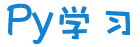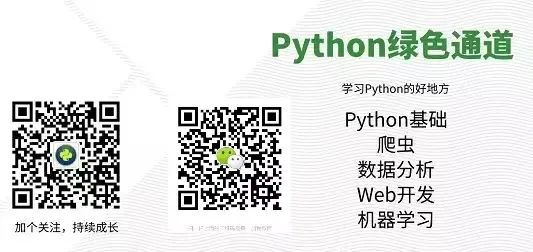注册    登录创作新主题

Python
 python开源   Django   Python   DjangoApp   pycharm
DATA
 docker   Elasticsearch

 问与答   闲聊   招聘   翻译   创业   分享发现   分享创造   求职   区块链   支付之战
aigc
 aigc   chatgpt
WEB开发
 linux   MongoDB   Redis   DATABASE   NGINX   其他Web框架   web工具   zookeeper   tornado   NoSql   Bootstrap   js   peewee   Git   bottle   IE   MQ   Jquery

Python88.com
 反馈   公告   社区推广

Py学习  »  Python

# 20 个非常有用的 Python 单行代码！

Python绿色通道 • 8 月前 • 110 次点击

```后台回复【大礼包】送你Python自学大礼包```

## 1 一行 For 循环

for 循环是一个多行语句，但是在 Python 中，我们可以使用列表推导式方法在一行中编写 for 循环。以过滤小于250的值为例，查看下面的代码示例。

``#For循环在一行mylist = [200, 300, 400, 500]#正常方式result = [] ``for x in mylist:     if x > 250:         result.append(x) print(result) # [300, 400, 500]``#一行代码方式result = [x for x in mylist if x > 250] print(result) # [300, 400, 500]``

## 2 一行 While 循环

``#方法 1 Single Statement while True: print(1) #infinite 1#方法 2 多语句x = 0 while x 5: print(x); x= x + 1 # 0 1 2 3 4 5``

## 3 一行 IF Else 语句

``#if Else 在一行中#Example 1 if elseprint("Yes") if 8 > 9 else print("No") # No#Example 2 if elif else E = 2 print("High") if E == 5 else print("数据STUDIO") if E == 2 else print("Low") # 数据STUDIO  #Example 3 only ifif 3 > 2: print("Exactly") # Exactly``

## 4 一行合并字典

``# 在一行中合并字典d1 = { 'A': 1, 'B': 2 } d2 = { 'C': 3, 'D': 4 }#方法 1 d1.update(d2) print(d1) # {'A': 1, 'B': 2, 'C': 3, 'D': 4}#方法 2 d3 = {**d1, **d2} print(d3) # {'A': 1, 'B': 2, 'C': 3, 'D': 4}``

## 5 一行函数

``#函数在一行中#方法一def fun(x): return True if x % 2 == 0 else False print(fun(2)) # False#方法2fun = lambda x : x % 2 == 0 print(fun(2)) # True print(fun(3)) # False``

## 6 一行递归

``````# 单行递归#Fibonaci 单行递归示例def Fib(x): return 1 if x in {0

, 1} else Fib(x-1) + Fib(x-2)print(Fib(5)) # 8print(Fib(15)) # 987``````

## 7 一行数组过滤

Python 列表可以通过使用列表推导方法在一行代码中进行过滤。以过滤偶数列表为例。

``# 一行中的数组过滤mylist = [2, 3, 5, 8, 9, 12, 13, 15]#正常方式result = [] for x in mylist:     if x % 2 == 0:         result.append(x)print(result) # [2, 8, 12]# 单线方式result = [x for x in mylist if x % 2 == 0] print(result) # [2, 8, 12]``

## 8 一行异常处理

``# 一行异常处理#原始方式try:    print(x)except:    print("Error")#单行方式exec('try:print(x) \nexcept:print("Error")') # 错误``

## 9 一行列表转字典

``# 字典在一行mydict = ["John", "Peter", "Mathew", "Tom"]mydict = dict(enumerate(mydict))print(mydict) # {0: 'John', 1: 'Peter', 2: 'Mathew', 3: 'Tom'}``

## 10 一行多变量

Python 允许在一行中进行多个变量赋值。下面的示例代码将向你展示如何做到这一点。

``#多行变量#正常方式x = 5 y = 7 z = 10 print(x , y, z) # 5 7 10#单行方式a, b, c = 5, 7, 10 print(a, b, c) # 5 7 10``

## 11 一行交换值

``#换成一行#正常方式v1 = 100v2 = 200temp = v1v1 = v2 v2 = tempprint(v1, v2) # 200 100# 单行交换v1, v2 = v2, v1 print(v1, v2) # 200 100``

## 12 一行排序

``# 在一行中排序mylist = [32, 22, 11, 4, 6, 8, 12] # 方法 1mylist.sort() print(mylist) # # [4, 6, 8, 11, 12, 22, 32]print(sorted(mylist)) # [4, 6, 8, 11, 12, 22, 32]``

## 13 一行读取文件

``#一行读取文件#正常方式with open("data.txt", "r") as file:     data = file.readline()     print(data) # Hello world#单行方式data = [line.strip() for line in open("data.txt","r")] print(data) # ['hello world', 'Hello Python']``

## 14 一行类

``# 一行中的类#普通方式class Emp:     def __init__(self, name, age):         self.name = name         self.age = age        emp1 = Emp("云朵君", 22) print(emp1.name, emp1.age) # 云朵君 22#单行方式#方法 1 带有动态 Artibutes 的 LambdaEmp = lambda:None; Emp.name = "云朵君"; Emp.age = 22print(Emp.name, Emp.age) # 云朵君 22#方法 2 from collections import namedtupleEmp = namedtuple('Emp', ["name", "age"]) ("云朵君", 22) print(Emp.name, Emp.age) # 云朵君 22``

## 15 一行分号

``# 一行分号# 例 1 a = "Python"; b = "编程"; c = "语言"; print(a, b, c)# 输出# Python 编程语言``

## 16 一行打印

``# 一行打印#正常方式for x in range(1, 5):    print(x) # 1 2 3 4#单行方式print(*range(1, 5)) # 1 2 3 4print(*range(1, 6)) # 1 2 3 4 5``

## 17 一行map函数

Map 函数是适用的高阶函数。这将函数应用于每个元素。下面是我们如何在一行代码中使用 map 函数的示例。

``#在一行中mapprint(list(map(lambda a: a + 2, [5, 6, 7, 8, 9, 10])))# 输出# [7, 8, 9, 10, 11, 12]``

## 18 删除列表第一行中的 Mul 元素

``# 删除一行中的Mul元素mylist = [100, 200, 300, 400, 500]del mylist[1::2]print(mylist) # [100, 300, 500]``

## 19 一行打印图案

``# 在一行中打印图案# # 正常方式for x in range(3):    print('😀')# 输出# 😀 😀 😀#单行方式print('😀' * 3) # 😀 😀 😀 print('😀' * 2) # 😀 😀 print('😀' * 1) # 😀``

## 20 一行查找质数

``# 查找质数print(list(filter(lambda a: all(a % b != 0 for b in range(2, a)),                  range(2,20))))# 输出# [2, 3, 5, 7, 11, 13, 17, 19]``

`GPT-4 发布！ChatGPT 大升级！太太太太强了！重磅！微软再扔AI聊天画图炸弹！图文版ChatGPT诞生了`

Python社区是高质量的Python/Django开发社区